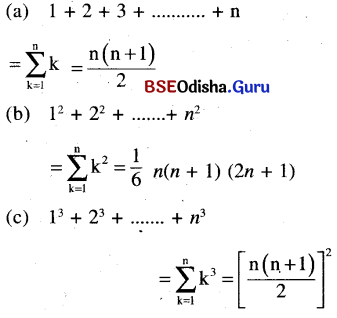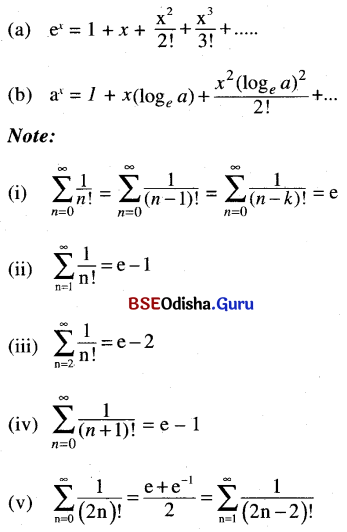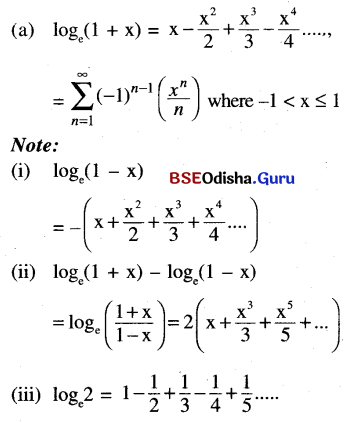# CHSE Odisha Class 11 Math Notes Chapter 10 Sequences and Series

Odisha State Board CHSE Odisha Class 11 Math Notes Chapter 10 Sequences and Series will enable students to study smartly.

## CHSE Odisha 11th Class Math Notes Chapter 10 Sequences and Series

Sequence:
A sequence is a function whose domain is N (The set of natural numbers).
Note: We can use the set of whole numbers as a domain.

Real Sequence:
If the range of a sequence is a subset of ‘R’, then it is a real sequence.
⇒ If: N → R is a sequence then f(n) for n = 1, 2, 3, ….. are the terms of the sequence.

Finite and infinite sequence:
A sequence with a finite number of terms is a finite sequence otherwise it is infinite.
Note: We denote a sequence by (tn) or {tn} where f(n) = tn

Series:
An expression of the type t1 + t2 + t3 + ….. (or ∑tn) where tn is the nth term of a sequence is a series.

Partial sums:
If $$\sum_{n=1}^{\infty} t_n$$ is a series then a sum $$\mathrm{S}_n=\sum_{k=1}^n t_k$$ is called the nth partial sum of the series for n = 1, 2, 3 …..
∴ s1 = t1, s2 = t1 + t2, s3 = t1 + t2 + t3 and so on.Progression:
Progression is a sequence whose terms follow as pattern.
Arithmetic progression (A.P):
A sequence  (tn) is an A.P. If tn+1 – tn = d (constant) for n = 1, 2, 3, …..

(a) General form: a, a + d, a + 2d, a + 3d …..
(b) nth term: tn = a + (n – 1)d, where t1 = a, and the common difference = d
(c) Sum of first n terms (Sn):
Sn = $$\frac{n}{2}$$[2a + (n – 1)d]
= $$\frac{n}{2}$$[a + l]
where a = first term
d = common difference
l = last term (or nth term)

Note:

1. If a, b, c are in A.P. then 2b = a + c.
2. If 3 numbers are in A.P. then we take them as a – d, a, a + d.
3. If 4 numbers are in A.P. then we take rhem as a – 3d, a – d, a + d, a + 3d.

(d) Insertion of arithmetic means between two given number:
Let m1, m2, m3 …. mn are ‘n’ arithmetic means between ‘a’ and ‘b’ then mk = a + $$\frac{k(b-a)}{n+1}$$ for k = 1, 2, ….. n.

Geometric progression (G.P):
If $$\frac{t_{n+1}}{t_n}$$ = r (constant), for n = 1, 2, 3, ….. then the sequence (tn) is a geometrical progression.

(a) General form: a, ar, ar2, ar3 …..
(b) nth term of GP: nth term of G.P. = tn = arn-1.
(c) sum of first n terms of a G.P.: Sn = $$\frac{a\left(1-r^n\right)}{1-r}(\text { for } r \neq 1)$$
(d) sum of an infinite G.P.: If |r| < 1 then the sum of the infinite G.P. a, ar, ar2 ….. is S = $$\frac{a}{1-r}$$

Note:

1. If a, b, c are in G.P. then b2 = ac
2. If 3 numbers are in G.P. we take them as $$\frac{a}{r}$$, a, ar.
3. If 4 numbers are in G.P. then we take them as $$\frac{a}{r^3}, \frac{a}{r}, a r, a r^3$$

(e) Insertion of  geometric means between  two numbers:
If g1, g2 ……gn are n geometric means between a and b then gk = a $$\left(\frac{b}{a}\right)^{\frac{k}{n+1}}$$, k = 1, 2, 3, …. nHarmonic Progression (H.P):
A sequence a1, a2, a3 ….. of non zero numbers is called a Harmonic progression if the sequence $$\frac{1}{a_1}, \frac{1}{a_2}, \frac{1}{a_3}$$ ….. is an A.P.

(a) Harmonic mean:
Harmonic mean(H) between two numbers a and b is $$\frac{1}{\mathrm{H}}=\frac{\left(\frac{1}{a}+\frac{1}{b}\right)}{2}$$
= $$\frac{a+b}{2 a b}$$
⇒ H = $$\frac{2 a b}{a+b}$$

(b) Insertion of n harmonic means between two numbers:
Let H1, H2 ….. Hn are n harmonic means between a and b then $$\frac{1}{\mathrm{H}_K}=\frac{1}{a}$$ + kD, where D = $$\frac{a-b}{(n+1) a b}$$.

Relation among A.M., G.M. and H.M.
AM ≥ GM ≥ HM

Arithmetic co-geometric sequence(AGP):
If (an) is an A.P. and (bn) is an G.P. then the series (anbn) is called an arithmetic co-geometric sequence.

(a) General form: a, (a + d) r, (a + 2d) r2, (a + 3d)r3,…..
(b) nth term of A.G.P.: tn = {a + (n – 1)d} rn-1.
(c) sum of first terms of A.G.P.:
The sum of first n terms of A.G.P. a, (a + d) r, (a + 2d) r2, …..  is
Sn = $$\frac{a}{1-r}+d r\left(\frac{1-r^{n-1}}{(1-r)^2}\right)-\frac{[a+(n-1) d] r^n}{1-r}$$ for r ≠ 1
(d) sum of infinite A.G.P.: If |r| < 1 then we have $$S_{\infty}=\frac{a}{1-r}+\frac{d r}{(1-r)^2}$$

Sum of special sequences.:Binomial Series:
(a) Binomial theorem for any real index:

• (1 + x) n = 1 + nx + $$\frac{n(n-1)}{2} x^2+\frac{n(n-1)(n-2)}{3 !} x^3+\ldots$$ for |x| < 1
• (1 – x)-1 = 1 + x + x2 …..
• (1 + x)-1 = 1 – x + x2 – x3 …..
• (1 + x)-2 = 1 – 2x + 3x2 – 4x3 …..
• (1 – x)-2 = 1 + 2x + 3x2 + …..Exponential series:Logarithmic Series: